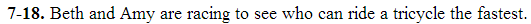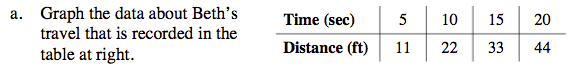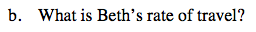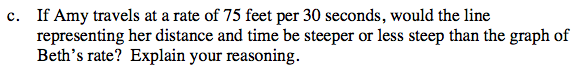### Home > MC2 > Chapter 7 > Lesson 7.1.2 > Problem7-18

7-18.
1. Beth and Amy are racing to see who can ride a tricycle the fastest. 7-18 HW eTool (CPM). Homework Help ✎

1. Graph the data about Beth's travel that is recorded in the table below.

 Time (sec) Distance (ft) 5 10 15 20 11 22 33 44
2. What is Beth's rate of travel?

3. If Amy travels at a rate of 75 feet per 30 seconds, would the line representing her distance and time be steeper or less steep than the graph of Beth's rate? Explain your reasoning.Time should be on the x-axis.Rate is distance divided by time.

2.2 feet per secondFind the number of feet Amy would travel per second.
Is it larger than your answer for part (b)?

$\frac{75 feet}{30 seconds} = 2.5 \frac{feet}{second}$

If you are still confused about which line should be steeper, try plotting
a line on your graph from part (a) to represent Amy's rate of travel.
The line should pass through the point (0, 0) and have a slope of 2.5.

Use the eTool below to graph the data about Beth's travel.
Click the link at right for the full version of the eTool: MC2 7-18 HW eTool